# comm.OFDMModulator

Modulate signal using OFDM method

## Description

The `OFDMModulator` object modulates a signal using the orthogonal frequency division multiplexing method. The output is a baseband representation of the modulated signal.

To modulate a signal using OFDM:

1. Create the `comm.OFDMModulator` object and set its properties.

2. Call the object with arguments, as if it were a function.

To learn more about how System objects work, see What Are System Objects?

## Creation

### Syntax

``hMod = comm.OFDMModulator``
``hMod = comm.OFDMModulator(Name,Value)``
``hMod = comm.OFDMModulator(hDemod)``

### Description

example

````hMod = comm.OFDMModulator` creates an OFDM modulator System object™.```

example

````hMod = comm.OFDMModulator(Name,Value)` specifies Properties using one of more name-value pair arguments. Enclose each property name in quotes. For example, `comm.OFDMModulator('NumSymbols',8)` specifies eight OFDM symbols in the time-frequency grid.```

example

````hMod = comm.OFDMModulator(hDemod)` sets the OFDM modulator system object properties based on the specified OFDM demodulator system object `comm.OFDMDemodulator`.```

## Properties

expand all

Unless otherwise indicated, properties are nontunable, which means you cannot change their values after calling the object. Objects lock when you call them, and the `release` function unlocks them.

If a property is tunable, you can change its value at any time.

For more information on changing property values, see System Design in MATLAB Using System Objects.

Number of Fast Fourier Transform (FFT) points, specified as a positive integer. The length of the FFT, NFFT, must be greater than or equal to 8 and is equivalent to the number of subcarriers.

Data Types: `double`

Number of subcarriers allocated to the left and right guard bands, specified as a two-element column vector of integers. The number of subcarriers must fall within [0, NFFT/2⌋ − 1]. This vector has the form [NleftG, NrightG], where NleftG and NrightG specify the left and right guard bands, respectively.

Data Types: `double`

Option to insert DC null, specified as a numeric or logical `0` (`false`) or `1` (`true`). The DC subcarrier is the center of the frequency band and has the index value:

• (`FFTLength` / 2) + 1 when `FFTLength` is even

• (`FFTLength` + 1) / 2 when `FFTLength` is odd

Option to specify pilot input, specified as a numeric or logical `0` (`false`) or `1` (`true`). If this property is `1` (`true`), you can assign individual subcarriers for pilot transmission. If this property is `0` (`false`), pilot information is assumed to be embedded in the input data.

Pilot subcarrier indices, specified as a column vector. If the PilotCarrierIndices property is set to `1` (`true`), you can specify the indices of the pilot subcarriers. You can assign the indices to the same or different subcarriers for each symbol. Similarly, the pilot carrier indices can differ across multiple transmit antennas. Depending on the desired level of control for index assignments, the dimensions of the property vary. Valid pilot indices fall in the range

`$\left[{N}_{\text{leftG}}+1,\text{\hspace{0.17em}}{N}_{\text{FFT}}/2\right]\cup \left[{N}_{\text{FFT}}/2+2,\text{\hspace{0.17em}}{N}_{\text{FFT}}-{N}_{\text{rightG}}\right],$`

where the index value cannot exceed the number of subcarriers. When the pilot indices are the same for every symbol and transmit antenna, the property has dimensions Npilot-by-1. When the pilot indices vary across symbols, the property has dimensions Npilot-by-Nsym. If you transmit only one symbol but multiple transmit antennas, the property has dimensions Npilot-by-1-by-Nt., where Nt. is the number of transmit antennas. If the indices vary across the number of symbols and transmit antennas, the property has dimensions Npilot-by-Nsym-by-Nt. If the number of transmit antennas is greater than one, ensure that the indices per symbol must be mutually distinct across antennas to minimize interference.

To enable this property, set the `PilotInputPort` property to `1` (`true`).

Length of cyclic prefix, specified as a positive integer. If you specify a scalar, the prefix length is the same for all symbols through all antennas. If you specify a row vector of length Nsym, the prefix length can vary across symbols but remains the same through all antennas.

Data Types: `double`

Option to apply raised cosine window between OFDM symbols, specified as `true` or `false`. Windowing is the process in which the OFDM symbol is multiplied by a raised cosine window before transmission to more quickly reduce the power of out-of-band subcarriers. Windowing reduces spectral regrowth.

Length of raised cosine window, specified as a positive scalar. This value must be less than or equal to the minimum cyclic prefix length. For example, in a configuration of four symbols with cyclic prefix lengths 12, 14, 16, and 18, the window length must be less than or equal to 12.

To enable this property, set the `Windowing` property to `1` (`true`).

Number of OFDM symbols in the time-frequency grid, specified as a positive integer.

Number of transmit antennas, used to transmit the OFDM modulated signal, specified as a positive integer.

## Usage

### Syntax

``waveform = hMod(insignal)``
``waveform = hMod(data,pilot)``

### Description

````waveform = hMod(insignal)` applies OFDM modulation the specified baseband signal and returns the modulated OFDM baseband signal.```
````waveform = hMod(data,pilot)` assigns the pilot signal, `pilot`, into the frequency subcarriers specified by the PilotCarrierIndices property value of the `hMod` system object. To enable this syntax set the PilotCarrierIndices property to `true`.```

### Input Arguments

expand all

Input baseband signal, specified as a matrix or 3-D array of numeric values. The input baseband signal must be of size Nf-by-Nsym-by-Nt. where Nf is the number of frequency subcarriers excluding guard bands and DC null.

Data Types: `double`
Complex Number Support: Yes

Input data, specified as a matrix or 3-D array. The input must be a numeric of size Nd-by-Nsym-by-Nt. where Nd is the number of data subcarriers in each symbol. For more information on how Nd is calculated, see the PilotCarrierIndices property.

Data Types: `double`
Complex Number Support: Yes

Pilot signal, specified as a 3-D array of numeric values. The pilot signal must be of size Npilot-by-Nsym-by-Nt.

Data Types: `double`
Complex Number Support: Yes

### Output Arguments

expand all

OFDM Modulated baseband signal, returned as a 2-D array. If the `CyclicPrefixLength` property is a scalar, the output `waveform` is of size ((NFFT+CPlen)⁎Nsym)-by-Nt. Otherwise, the size is (NFFT⁎Nsym+∑(CPlen))-by-Nt.

Data Types: `double`
Complex Number Support: Yes

## Object Functions

To use an object function, specify the System object as the first input argument. For example, to release system resources of a System object named `obj`, use this syntax:

`release(obj)`

expand all

 `info` Provide dimensioning information for OFDM modulator `showResourceMapping` Show the subcarrier mapping of the OFDM symbols created by the OFDM modulator System object
 `step` Run System object algorithm `release` Release resources and allow changes to System object property values and input characteristics `reset` Reset internal states of System object

## Examples

collapse all

Create and display an OFDM modulator System object™ with default property values.

`hMod = comm.OFDMModulator`
```hMod = comm.OFDMModulator with properties: FFTLength: 64 NumGuardBandCarriers: [2x1 double] InsertDCNull: false PilotInputPort: false CyclicPrefixLength: 16 Windowing: false NumSymbols: 1 NumTransmitAntennas: 1 ```

Modify the number of subcarriers and symbols.

```hMod.FFTLength = 128; hMod.NumSymbols = 2;```

Verify that the number of subcarriers and the number of symbols changed.

`disp(hMod)`
``` comm.OFDMModulator with properties: FFTLength: 128 NumGuardBandCarriers: [2x1 double] InsertDCNull: false PilotInputPort: false CyclicPrefixLength: 16 Windowing: false NumSymbols: 2 NumTransmitAntennas: 1 ```

Use the `showResourceMapping` object function to show the mapping of data, pilot, and null subcarriers in the time-frequency space.

`showResourceMapping(hMod)`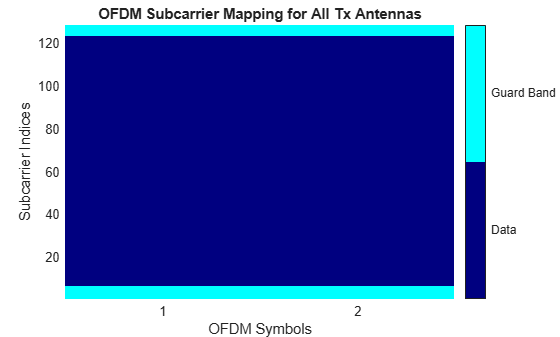Create an OFDM demodulator System object™ with default property values. Then, specify pilot indices for a single symbol and two transmit antennas.

Setting the `PilotCarrierIndices` property of the demodulator affects the number of transmit antennas in the OFDM modulator when you use the demodulator in the creation of the modulator. The number of receive antennas in the demodulator is uncorrelated with the number of transmit antennas.

```ofdmDemod = comm.OFDMDemodulator; ofdmDemod.PilotOutputPort = true; ofdmDemod.PilotCarrierIndices = ... cat(3,[12; 26; 40; 54],[13; 27; 41; 55]);```

Use the OFDM demodulator to construct the OFDM modulator.

`ofdmMod = comm.OFDMModulator(ofdmDemod);`

Display the properties of the OFDM modulator and demodulator, verifying that the applicable properties match.

`disp(ofdmMod)`
``` comm.OFDMModulator with properties: FFTLength: 64 NumGuardBandCarriers: [2x1 double] InsertDCNull: false PilotInputPort: true PilotCarrierIndices: [4x1x2 double] CyclicPrefixLength: 16 Windowing: false NumSymbols: 1 NumTransmitAntennas: 2 ```
`disp(ofdmDemod)`
``` comm.OFDMDemodulator with properties: FFTLength: 64 NumGuardBandCarriers: [2x1 double] RemoveDCCarrier: false PilotOutputPort: true PilotCarrierIndices: [4x1x2 double] CyclicPrefixLength: 16 NumSymbols: 1 NumReceiveAntennas: 1 ```

The `showResourceMapping` method displays the time-frequency resource mapping for each transmit antenna.

Construct an OFDM modulator.

`mod = comm.OFDMModulator;`

Apply the `showResourceMapping` method.

`showResourceMapping(mod)`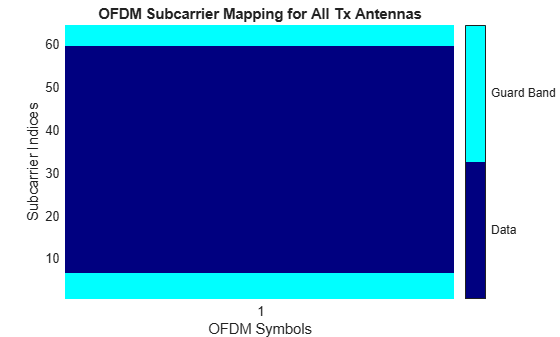Insert a DC null.

`mod.InsertDCNull = true;`

Show the resource mapping after adding the DC null.

`showResourceMapping(mod)`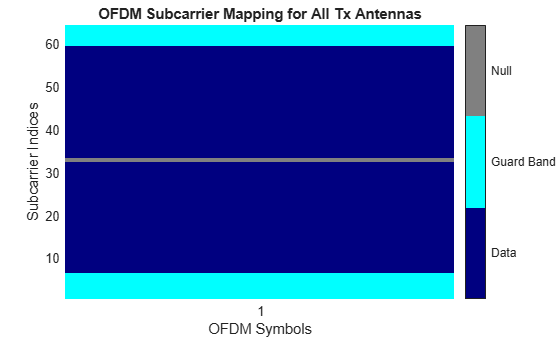Create an OFDM modulator and specify the subcarrier indices for the pilot signals. Specify the indices for each symbol and transmit antenna. When the number of transmit antennas is greater than one, set different pilot indices for each symbol between antennas.

Create an OFDM modulator System object, specifying two symbols and inserting a DC null.

```mod = comm.OFDMModulator('FFTLength',128,'NumSymbols',2,... 'InsertDCNull',true);```

Enable the pilot input port so you can specify the pilot indices.

`mod.PilotInputPort = true;`

Specify the same pilot indices for both symbols.

`mod.PilotCarrierIndices = [12; 56; 89; 100];`

Visualize the placement of the pilot signals and nulls in the OFDM time-frequency grid by using the `showResourceMapping` object function.

`showResourceMapping(mod)`Specify different indices for the second symbol by concatenating a second column of pilot indices to the `PilotCarrierIndices` property.

```mod.PilotCarrierIndices = cat(2,mod.PilotCarrierIndices, ... [17; 61; 94; 105]);```

Verify that the pilot subcarrier indices differ between the two symbols.

`showResourceMapping(mod)`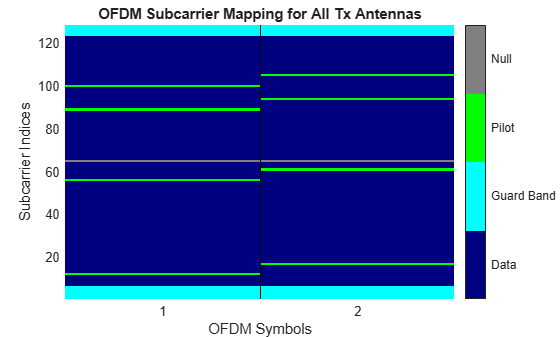Increase the number of transmit antennas to two.

`mod.NumTransmitAntennas = 2;`

Specify the pilot indices for each of the two transmit antennas. To provide indices for multiple antennas while minimizing interference among the antennas, set the `PilotCarrierIndices` property as a 3-D array such that the indices for each symbol differ among antennas.

`mod.PilotCarrierIndices = cat(3,[20; 50; 70; 110], [15; 60; 75; 105]);`

Display the resource mapping for the two transmit antennas. The gray lines denote the insertion of custom nulls. The nulls are created by the object to minimize interference among the pilot symbols from different antennas.

`showResourceMapping(mod)`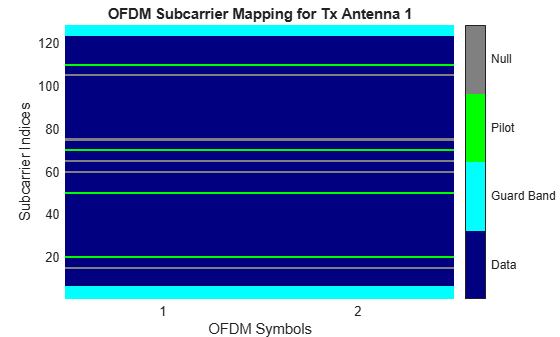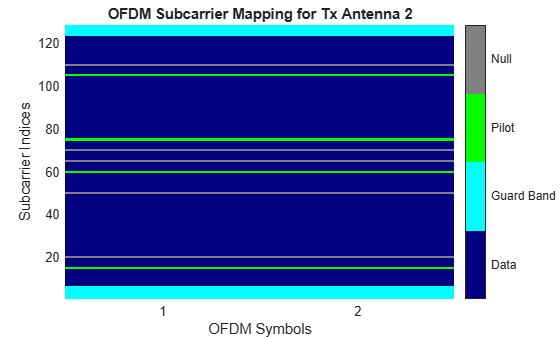Specify the length of the cyclic prefix for each OFDM symbol.

Create an OFDM modulator, specifying five symbols, four left and three right guard-band subcarriers, and the cyclic prefix length for each OFDM symbol.

```mod = comm.OFDMModulator('NumGuardBandCarriers',[4;3],... 'NumSymbols',5,... 'CyclicPrefixLength',[12 10 14 11 13]);```

Display the properties of the OFDM modulator, verifying that the cyclic prefix length changes across symbols.

`disp(mod)`
``` comm.OFDMModulator with properties: FFTLength: 64 NumGuardBandCarriers: [2x1 double] InsertDCNull: false PilotInputPort: false CyclicPrefixLength: [12 10 14 11 13] Windowing: false NumSymbols: 5 NumTransmitAntennas: 1 ```

Get the OFDM modulator data dimensions by using the `info` object function.

Construct an OFDM modulator System object™ with user-specified pilot indices, an inserted DC null, and specify two transmit antennas.

```hMod = comm.OFDMModulator('NumGuardBandCarriers',[4;3], ... 'PilotInputPort',true, ... 'PilotCarrierIndices',cat(3,[12; 26; 40; 54], ... [11; 25; 39; 53]), ... 'InsertDCNull',true, ... 'NumTransmitAntennas',2);```

Use the `info` object function to get the modulator input data, pilot input data, and output data sizes.

`info(hMod)`
```ans = struct with fields: DataInputSize: [48 1 2] PilotInputSize: [4 1 2] OutputSize: [80 2] ```

Generate OFDM modulated symbols for use in link-level simulations.

Construct an OFDM modulator with an inserted DC null, seven guard-band subcarriers, and two symbols having different pilot indices for each symbol.

```mod = comm.OFDMModulator( ... 'NumGuardBandCarriers',[4;3], ... 'PilotInputPort',true, ... 'PilotCarrierIndices',[12 11; 26 27; 40 39; 54 55], ... 'NumSymbols',2, ... 'InsertDCNull',true);```

Determine input data, pilot, and output data dimensions.

`modDim = info(mod);`

Generate random data symbols for the OFDM modulator. The structure variable, `modDim`, determines the number of data symbols.

```dataIn = complex( ... randn(modDim.DataInputSize),randn(modDim.DataInputSize));```

Create a pilot signal that has the correct dimensions.

```pilotIn = complex( ... rand(modDim.PilotInputSize),rand(modDim.PilotInputSize));```

Apply OFDM modulation to the data and pilot signals.

`modData = mod(dataIn,pilotIn);`

Use the OFDM modulator object to create the corresponding OFDM demodulator.

`demod = comm.OFDMDemodulator(mod);`

Demodulate the OFDM signal and output the data and pilot signals.

`[dataOut, pilotOut] = demod(modData);`

Verify that, within a tight tolerance, the input data and pilot symbols match the output data and pilot symbols.

```isSame = (max(abs([dataIn(:) - dataOut(:); ... pilotIn(:) - pilotOut(:)])) < 1e-10)```
```isSame = logical 1 ```